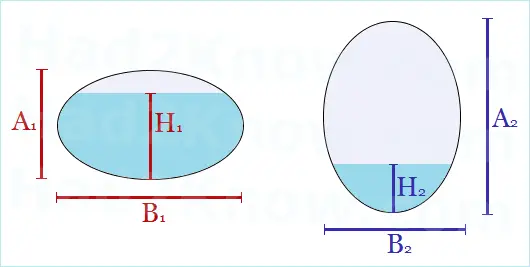# Area of an Ellipse Segment

### Elliptical Tank Volume Calculator

Elliptical Tank Volume and Segment Area
Tank Height A =
Tank Width B =
Segment Height (Water Depth) H =
Tank Length L =

Segment Area =
Tank Volume =

Many industrial storage tanks are in the shape of elliptical cylinders, for instance, oil tankers. One of the most commonly encountered math problem in tank geometry is figuring the volume of water in a partially filled tank that rests on its side. To compute the volume, you must find the area of the cross-sectional shape (an elliptical segment) and then multiply it by the length of the tank.

Computing the area of an ellipse segment is easy so long as you know the tank's dimensions, the height of the segment, and of course the right area formula. You can reference the equation given below, or use the convenient tank volume and area calculator on the left.

The good news about calculating the area of an elliptical segment is that it doesn't matter if the oval is tall and skinny or flat and wide, or if the water level is less than half or more than half of the tank's height--one equation covers every case.If the height of the tank is A, the width of the tank B, and the height of the elliptical segment h then the area of the segment is given by the equation:

area = (AB/4)[arccos(1 - 2h/A) - (1 - 2h/A)sqrt(4h/A - 4h2/A2)],

where arccos is in radians, not degrees. If the tank has a length of L, then the corresponding volume formula is simply:

volume = (ABL/4)[arccos(1 - 2h/A) - (1 - 2h/A)sqrt(4h/A - 4h2/A2)].

To find the maximum area or maximum volume in the tank, set h = A, that is, set the height of the liquid equal to the height of the tank. This gives you the elliptical area formula (pi/4)AB, and the elliptical tank volume formula (pi/4)ABL.

Example: Suppose an elliptical tank is 15 feet long, and 4 feet wide, and 3 feet tall when laid on its side. The tank is filled with water to a depth of 24 inches. Find the volume of water in the tank.

We have L = 15, A = 3, B = 4, and h = 2, since we must convert inches to feet. Plugging these values into the volume formula gives us

volume = (3*4*15/4)[arccos(1 - 4/3) - (1 - 4/3)sqrt(8/3 - 16/9)]
= 45[1.9106 + (1/3)sqrt(8/9)]
= 45[1.9106 + 0.3143]
= 100.1205 cubic feet.